## How to change or convert number to text in Excel?

Sometimes you may not want the numbers in the cells getting involved in calculating, or you may want to display leading zeros in numbers in cells. For doing so, you may need to change a number into text. The following methods can convert numbers in cells to text in Excel.

Convert number to text with Text function

Convert number to text with Format Cells command

#### Convert number to text with Text function

If you are familiar with Microsoft Excel's formulas, you can convert numbers in cells to text with Text function.

If you just only want to convert the number to text without any formatting, you can use the formula: =TEXT(A1,"0");

1. In cell E1, please enter the formula =TEXT(A1,"0").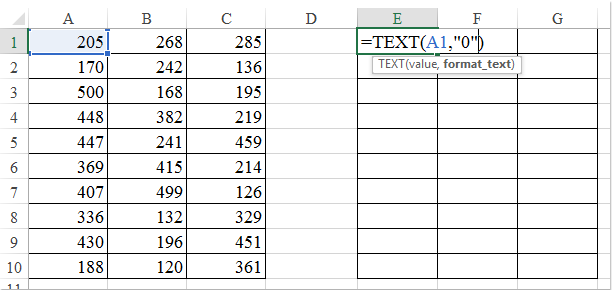2. Then press Enter key. And select the cell E1, drag the fill handle over the range of cells that you want to apply this formula.See screenshot: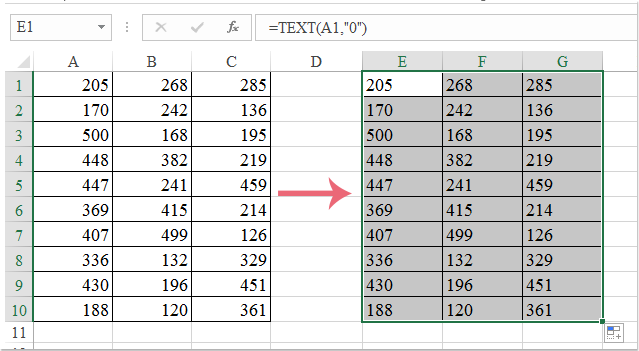Note: As the data is formula, you can change the formulas to values. Copy the cells and right-click where you want to paste the values and select Paste special > Values.

If you want to display the leading zero in numbers, you can use this formula: =TEXT(A1,"00000"); such as convert 23 to 00023.

As the above steps, if you apply this formula, you will get the following result: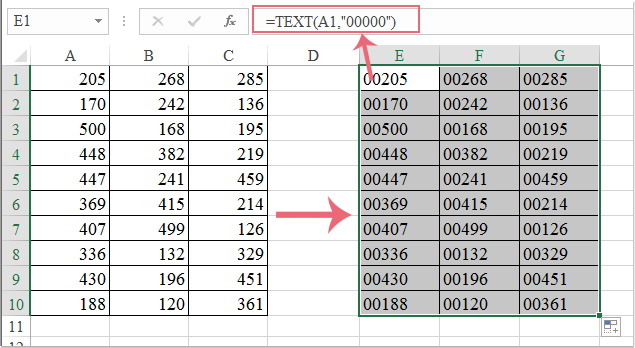And if you want to convert the numbers to text which keep several decimal digits, you can use this formula: =TEXT(A1,"0.000"). Such as convert 23 to 23.000.

Also with above steps, if you apply this formula, you will get the result as the following screenshots:Note: you can define the text style. For example, if you want to convert the numbers to text with a telephone number format, you can replace the "000" with "00-00000", or others.

 Convert or spell out numbers to English or Chinese currency: With Kutools for Excel's Numbers to Words feature, you can spell out numbers into English and Chinese words in Excel.For example, it can spell out the "12.75" to "Twelve dollars and seventy-five cents".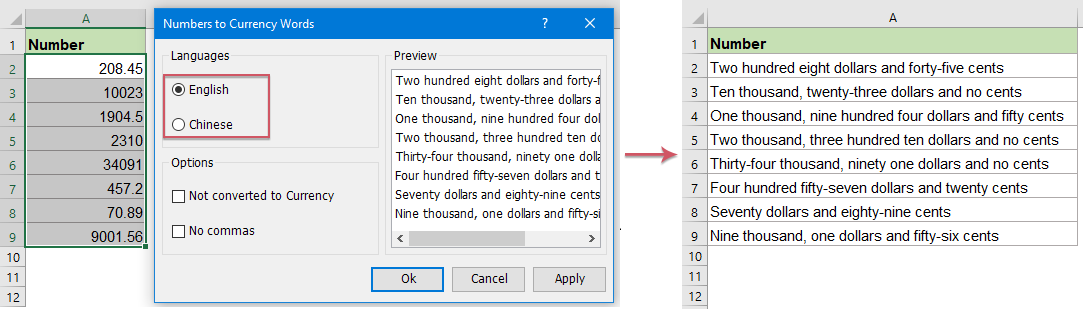Kutools for Excel: with more than 300 handy Excel add-ins, free to try with no limitation in 30 days.

#### Convert number to text with Format Cells command

Microsoft Excel's Format Cells command is also able to convert numbers to text.

1. Select the numbers that you want to convert to text.

2. Right click the selected range, and choose the Format Cells item from context menu. See screenshot: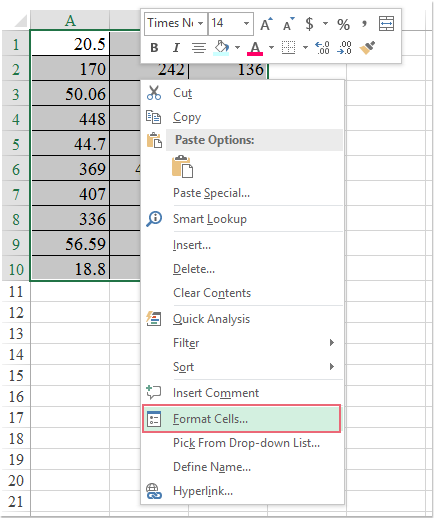3. In the Format Cells dialog box, select the Text item in the Category box under Number tab, and then click the OK button. See screenshot: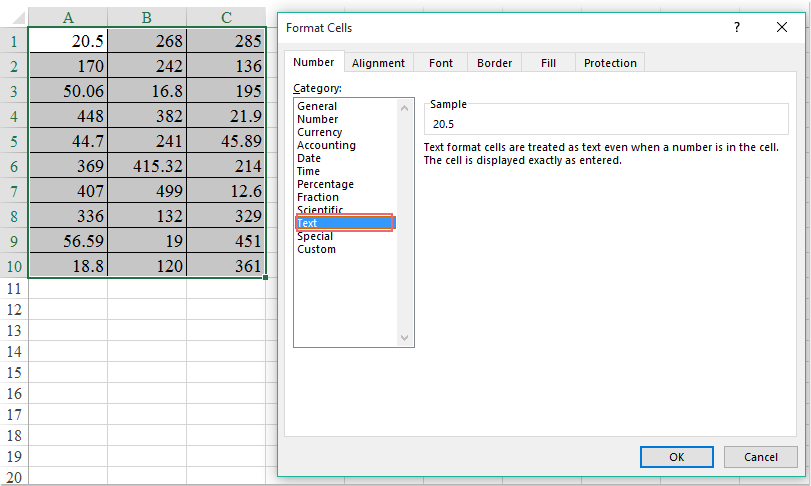4. Then numbers in selected range are converted into text.

#### Convert number to text with Kutools for Excel

If you have Kutools for Excel installed, it will be easier for you to convert numbers in cells to text with Convert between Text and Number.

 : with more than 300 handy Excel add-ins, free to try with no limitation in 30 days.

After installing Kutools for Excel, please do as below:

1. Select the range with numbers that you want to change to text.

2. Click Kutools > Content > Convert between Text and Number. See screenshot: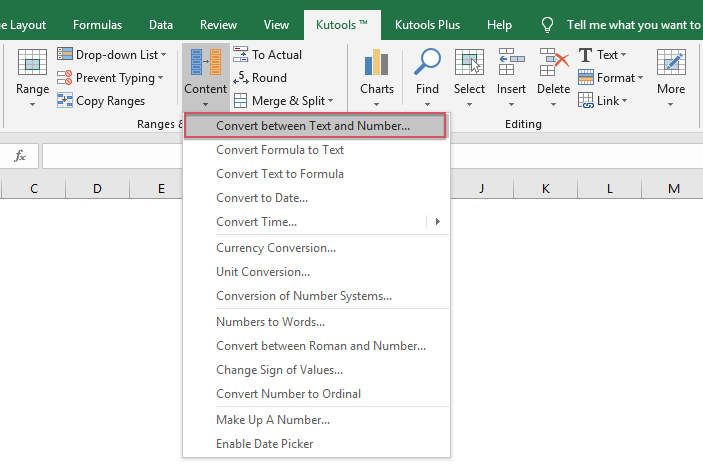3. In the Convert between Text and Number dialog box, check the Number to text option, and then click the OK or Apply button. And all numbers have been converted to text in the original range. See screenshot: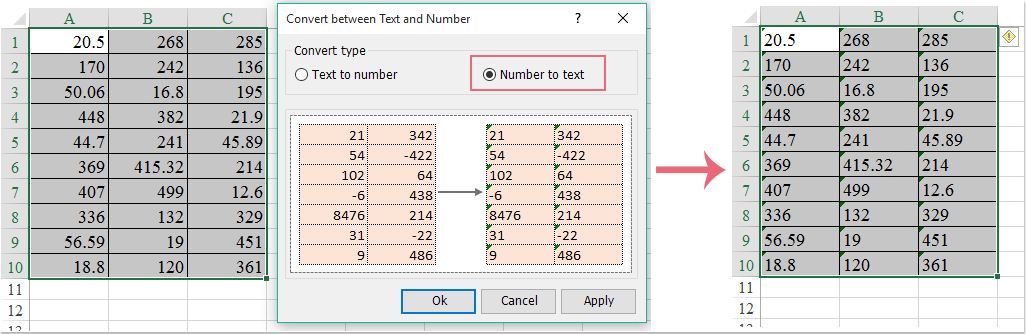Kutools for Excel: with more than 300 handy Excel add-ins, free to try with no limitation in 30 days.

Related article:

### Best Office Productivity Tools

Supercharge Your Spreadsheets： Experience Efficiency Like Never Before with Kutools for Excel

 Popular Features: Find/Highlight/Identify Duplicates   |  Delete Blank Rows   |  Combine Columns or Cells without Losing Data   |   Round without Formula ... Super Lookup: Multiple Criteria VLookup  |   Multiple Value VLookup  |   VLookup Across Multiple Sheets   |   Fuzzy Lookup .... Advanced Drop-down List: Quickly Create Drop Down List   |  Dependent Drop Down List   |  Multi-select Drop Down List .... Column Manager: Add a Specific Number of Columns   |   Move Columns   |   Unhide Columns   |   Compare Columns to Select Same & Different Cells ... Featured Features: Grid Focus   |  Design View   |   Big Formula Bar   |  Workbook & Sheet Manager   |  Resource Library (Auto Text)   |  Date Picker   |  Combine Worksheets   |  Encrypt/Decrypt Cells   |  Send Emails by List   |  Super Filter   |   Special Filter (filter bold/italic/strikethrough...) ... Top 15 Toolset:  12 Text Tools (Add Text, Remove Characters, ...)   |   50+ Chart Types (Gantt Chart, ...)   |   40+ Practical Formulas (Calculate age based on birthday, ...)   |   19 Insertion Tools (Insert QR Code, Insert Picture from Path, ...)   |   12 Conversion Tools (Numbers to Words, Currency Conversion, ...)   |   7 Merge & Split Tools (Advanced Combine Rows, Split Cells, ...)   |   Many More...

Kutools for Excel boasts over 300 features, ensuring that what you need is just a click away...

Supports Office/Excel 2007-2021 & newer, including 365   |   Available in 44 languages   |   Enjoy a full-featured 30-day free trial.#### Office Tab Brings Tabbed interface to Office, and Make Your Work Much Easier

• Enable tabbed editing and reading in Word, Excel, PowerPoint, Publisher, Access, Visio and Project.
• Open and create multiple documents in new tabs of the same window, rather than in new windows.
• Increases your productivity by 50%, and reduces hundreds of mouse clicks for you every day!No ratings yet. Be the first to rate!
This comment was minimized by the moderator on the site
Szeretnék egy olyat csinálni, hogy a különböző mezőkbe betűket írok és ezeknek a betűknek legyen szám értékük. Ezekkel műveleteket szeretnék végrehajtani. Pl.: sz=8 t=4 sz+t=12. Válaszát előre is köszönöm.
This comment was minimized by the moderator on the site
Hi there,

You can use the SUM function along with INDEX and MATCH: =SUM(INDEX(B2:B8,MATCH(A11:B11,A2:A8,0)))

In the formula, B2:B8 is the column that lists the values you assigned to the letters, A11:B11 is the letters you want to sum, A2:A8 is the column that lists all the letters.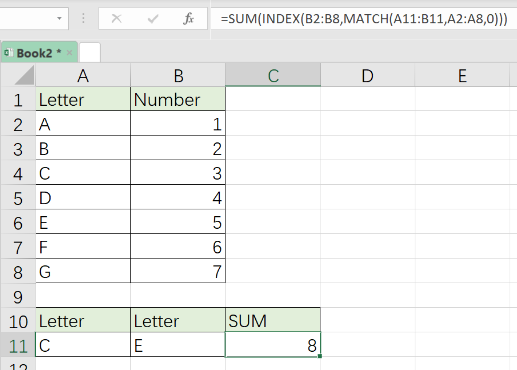Amanda
This comment was minimized by the moderator on the site
*****RESPUESTA A LA PREGUNTA DE COMO PASAR UN NUMERO A SU EXPRESION CON LETRAS (SIN MACRO)*****

Tenía construida una Macro para que realizara esa función, con la actualización de Office no pude mantenerla ya que no funcionan cuando están en línea solo en carpetas seguras, lo resolví creando una tabla siendo la primera columna el dato con el digito que queremos pasar a letra y en las siguientes columnas el numero en letra, lo realice según mi número más alto , en este caso empecé por DIEZ MIL, después otra columna con los cientos (CIENTO, DOCIENTOS, etc.), posteriormente los numero del 1 al 99 (VEINTIUNO, TREINTA, etc.;), también agregue una columna "Y" y los números del 1 al 9 (DOS, TRES, CUATRO, etc.) al final concatene todo y agregue detalles como mayúsculas, pesos, M.N y por supuesto espacios.

Para mandarlos a llamar utilice un BUSCARV con combinaciones que necesitaba, como ENTERO o DERECHA según lo que realices.

Quedando algo así:

=+SI(referencia de celda<2,SI.ERROR(CONCAT("( ",+BUSCARV(ENTERO(referencia de celda),hoja y columnas,10,0)," PESO ",DERECHA(DECIMAL(referencia de celda),2),"/100 M.N. )"),"( PESOS 00/100 M.N. )"),SI.ERROR(CONCAT("( ",+BUSCARV(ENTERO(referencia de celda),hoja y columnas,10,0)," PESOS ",DERECHA(DECIMAL(referencia de celda),2),"/100 M.N. )"),"( PESOS 00/100 M.N. )"))

oculte formulas y la hoja con mi tabla bloqueando hoja y libro.

Espero les ayude, esta laborioso pero la mayoría es rellenar datos, a mí me funciono.

Saludos!
This comment was minimized by the moderator on the site
How to translate a number into text in different languages on Excel ?

This comment was minimized by the moderator on the site
how do I maintain -1 as a superscript when I make a graph from an excel spreadsheet
This comment was minimized by the moderator on the site
I would like to convert the date 201901 in word " january¨ or number 01 (means january in numbers) in excel sheet. Thank you
This comment was minimized by the moderator on the site
How do you convert to text an amount with decimal number of which the decimal numbers remain as numbers? example: 750.25 - SEVEN HUNDRED FIFTY & 25/100.. Thank you
This comment was minimized by the moderator on the site
Large thanks!
This comment was minimized by the moderator on the site
Cuando convierto un numero en texto me lo pone en Ingles y en Dólares, lo necesito es en Español y en pesos Mexicanos, ¿como puedo resolver esto?
De antemano gracias por la atención que se sirva darme.
This comment was minimized by the moderator on the site
Hi, not working in our currency which is philippine peso. Kindly help. Thank you.
This comment was minimized by the moderator on the site
After converting words to figures, How can start with "Rupees" and end with "Only"
This comment was minimized by the moderator on the site
If i have assign
0 as Q
1 as W
2 as R
3 as E
4 as Z
5 as S
6 as T
7 as B
8 as K
9 as A
how to convert a numerical value to text code like 2356 i want 2 as R, 3 as E, 5 as S, 6 as T, so when i write 2356 i got result REST?
This comment was minimized by the moderator on the site
A workaround can be using the SUBSTITUTE formula ten times to replace all the numbers with the respective letters:

[span style="word-break: break-all"]=SUBSTITUTE(SUBSTITUTE(SUBSTITUTE(SUBSTITUTE(SUBSTITUTE(SUBSTITUTE(SUBSTITUTE(SUBSTITUTE(SUBSTITUTE(SUBSTITUTE(A1,0,"Q"),1,"W"),2,"R"),3,"E"),4,"Z"),5,"S"),6,"T"),7,"B"),8,"K"),9,"A")[/span]

Where A1 is the reference cell for the numeric values.
This comment was minimized by the moderator on the site
When I entered Numbers in Cell Eg : 150 the will written as One hundred and fifty
This comment was minimized by the moderator on the site
how translate staff id to text as "filled" and vice versa for nil staff ID to 'vacant"
This comment was minimized by the moderator on the site
i m a novice. text in cells read as 1234 5678 1234 i.e with a space bet each 4 digit clusters. how can i compress it as 123456781234 ?
This comment was minimized by the moderator on the site
=SUBSTITUTE(A1," ","")
Where A1 is reference cell for 1234 5678 1234
This comment was minimized by the moderator on the site
Great tip thanks. Now FYI this didn't work at first for me, as for some reason it didn't recognize the space.. but when I cut and pasted the "space" from the source (cut-and-pasted financial values from a web page) instead of pressing the space bar it worked fine.
This comment was minimized by the moderator on the site
It might be another Character that appears like the Space character. When you copy and paste data from external sources such as websites, unwanted characters will come across with different ASCII codes that may look like the Space character.

The above formula meant to remove only the Space character from your string. Space character means the value of the Char(32) on the ASCII values.

Best would be to modify the formula as =SUBSTITUTE(A1,CHAR(32),"")
This comment was minimized by the moderator on the site
Try Using =CONCATENATE("",LEFT(H20,4),MID(H20,6,4),RIGHT(H20,4))

Whereis H20 is reference cell for 1234 5678 1234
This comment was minimized by the moderator on the site
Use replace function. Highlight the cells you want to edit then Ctrl+H. Replace space to blank
This comment was minimized by the moderator on the site
I want to convert digit into words in indian language? How to do that?
This comment was minimized by the moderator on the site
Add this with below formulas in one

&CHOOSE(MID(TEXT(B3,"000000000.00"),5,1)+1,,," Twenty"," Thirty"," Forty"," Fifty"," Sixty"," Seventy"," Eighty"," Ninety")
&IF(--MID(TEXT(B3,"000000000.00"),5,1)<>1,CHOOSE(MID(TEXT(B3,"000000000.00"),6,1)+1,," One"," Two"," Three"," Four"," Five"," Six"," Seven"," Eight"," Nine"),CHOOSE(MID(TEXT(B3,"000000000.00"),6,1)+1," Ten"," Eleven"," Twelve"," Thirteen"," Fourteen"," Fifteen"," Sixteen"," Seventeen"," Eighteen"," Nineteen"))
&IF((--MID(TEXT(B3,"000000000.00"),4,1)+MID(TEXT(B3,"000000000.00"),5,1)+MID(TEXT(B3,"000000000.00"),6,1))=0,,IF(OR((--MID(TEXT(B3,"000000000.00"),7,1)+MID(TEXT(B3,"000000000.00"),8,1)+MID(TEXT(B3,"000000000.00"),9,1))=0,--MID(TEXT(B3,"000000000.00"),7,1)<>0)," Thousand "," Thousand and "))
&CHOOSE(MID(TEXT(B3,"000000000.00"),7,1)+1,,"One","Two","Three","Four","Five","Six","Seven","Eight","Nine")
&IF(--MID(TEXT(B3,"000000000.00"),7,1)=0,,IF(AND(--MID(TEXT(B3,"000000000.00"),8,1)=0,--MID(TEXT(B3,"000000000.00"),9,1)=0)," Hundred "," Hundred and "))&
CHOOSE(MID(TEXT(B3,"000000000.00"),8,1)+1,,,"Twenty ","Thirty ","Forty ","Fifty ","Sixty ","Seventy ","Eighty ","Ninety ")
&IF(--MID(TEXT(B3,"000000000.00"),8,1)<>1,CHOOSE(MID(TEXT(B3,"000000000.00"),9,1)+1,,"One","Two","Three","Four","Five","Six","Seven","Eight","Nine"),CHOOSE(MID(TEXT(B3,"000000000.00"),9,1)+1,"Ten","Eleven","Twelve","Thirteen","Fourteen","Fifteen","Sixteen","Seventeen","Eighteen","Nineteen"))

&" Euro & "&RIGHT(TEXT(B3,"000000000.00"),2)&"/100"
This comment was minimized by the moderator on the site
when add both these formulas one after the other, it worked perfectly.

good work. thanks a lot.
Naufdeen M. Siddeek from Kandy, Sri Lanka

As an improvement if can add text for cents also, it would be more better.
I will try by myself ...
This comment was minimized by the moderator on the site
=CHOOSE(LEFT(TEXT(B3,"000000000.00"))+1,,"One","Two","Three","Four","Five","Six","Seven","Eight","Nine")
&IF(--LEFT(TEXT(B3,"000000000.00"))=0,,IF(AND(--MID(TEXT(B3,"000000000.00"),2,1)=0,--MID(TEXT(B3,"000000000.00"),3,1)=0)," Hundred"," Hundred and "))
&CHOOSE(MID(TEXT(B3,"000000000.00"),2,1)+1,,,"Twenty ","Thirty ","Forty ","Fifty ","Sixty ","Seventy ","Eighty ","Ninety ")
&IF(--MID(TEXT(B3,"000000000.00"),2,1)<>1,CHOOSE(MID(TEXT(B3,"000000000.00"),3,1)+1,,"One","Two","Three","Four","Five","Six","Seven","Eight","Nine"),
CHOOSE(MID(TEXT(B3,"000000000.00"),3,1)+1,"Ten","Eleven","Twelve","Thirteen","Fourteen","Fifteen","Sixteen","Seventeen","Eighteen","Nineteen"))
&IF((--LEFT(TEXT(B3,"000000000.00"))+MID(TEXT(B3,"000000000.00"),2,1)+MID(TEXT(B3,"000000000.00"),3,1))=0,,IF(AND((--MID(TEXT(B3,"000000000.00"),4,1)+MID(TEXT(B3,"000000000.00"),5,1)+MID(TEXT(B3,"000000000.00"),6,1)+MID(TEXT(B3,"000000000.00"),7,1))=0,(--MID(TEXT(B3,"000000000.00"),8,1)+RIGHT(TEXT(B3,"000000000.00")))>0)," Million and "," Million "))
&CHOOSE(MID(TEXT(B3,"000000000.00"),4,1)+1,,"One","Two","Three","Four","Five","Six","Seven","Eight","Nine")
&IF(--MID(TEXT(B3,"000000000.00"),4,1)=0,,IF(AND(--MID(TEXT(B3,"000000000.00"),5,1)=0,--MID(TEXT(B3,"000000000.00"),6,1)=0)," Hundred"," Hundred and"))
This comment was minimized by the moderator on the site
Try Using This -

=IF(MID(K14,1,1)="1","One",IF(MID(K14,1,1)="2","Two",IF(MID(K14,1,1)="3","Three",IF(MID(K14,1,1)="4","Four",IF(MID(K14,1,1)="5","Five",IF(MID(K14,1,1)="6","Six",IF(MID(K14,1,1)="7","Seven",IF(MID(K14,1,1)="8","Eight",IF(MID(K14,1,1)="9","Nine",IF(MID(K14,1,1)="0","Zero",""))))))))))&" "&IF(RIGHT(K14,1)="1","One",IF(RIGHT(K14,1)="2","Two",IF(RIGHT(K14,1)="3","Three",IF(RIGHT(K14,1)="4","Four",IF(RIGHT(K14,1)="5","Five",IF(RIGHT(K14,1)="6","Six",IF(RIGHT(K14,1)="7","Seven",IF(RIGHT(K14,1)="8","Eight",IF(RIGHT(K14,1)="9","Nine",IF(RIGHT(K14,1)="0","Zero",""))))))))))

Instead of Using "One" "Two" "Three", You can change what ever you want against perticular No, also above code is for only upto two digits. you can extended it to any no by adding IF with MID formula.

K14 is reference cell for Number.
This comment was minimized by the moderator on the site
Using this formula, 99 would convert into 'Nine Nine'. Same goes for every other number (two digited). Seems the question was to how to spell a numerical value.
This comment was minimized by the moderator on the site
I couldn't covert number into million and billion \$ currency. Help me to because I am confused.
This comment was minimized by the moderator on the site
I want to convert dollar currency into million or billion format . I am confused do it. could you plz guide me.
This comment was minimized by the moderator on the site
Some clarification, when I try to convert below number it changes its format, eg. given below can you explain why this happen? other than zero it works fine

B64=890151200020,
H64=0
J64=TEXT(B64,"000 0000 0000 0 "&H64) it changes to "089 0151 2000 2 0" instead of "890 1512 0002 0 0"
This comment was minimized by the moderator on the site
TEXT(B64&H64,"000 0000 0000 0 0")
This comment was minimized by the moderator on the site
Perfect solution has been found. Full formulas could not be written due to space limitation. mail me at mukeshbrahmankar(gmail) for the file. File would be sent on a friendly note, no commercial thing. Regards
This comment was minimized by the moderator on the site
send me excel convertor file on
This comment was minimized by the moderator on the site
Any chace t change date in Text format, not keeping Date format? Ex. 1/1/2017 d/m/yyyy in the same way but text formatting?
This comment was minimized by the moderator on the site
Use cell formating. You can get the result as 1-Jan-2017
This comment was minimized by the moderator on the site
tnx for your solution be healthy
This comment was minimized by the moderator on the site
I cannot reproduce your result. When using the TEXT formula trying to convert a number to text, the resulting content was left-aligned but there was no green triangle, meaning the content was still a number, not text. Same result when using the menu command. No triangle. I use Excel 2010.
This comment was minimized by the moderator on the site
I cannot reproduce your results. When I used the first method trying to convert a number to text, the resulting number was left-aligned but there was not green triangle, meaning the content was still a number, not text. Same result with using the menu command. No green triangle. I'm using Excel 2010.
This comment was minimized by the moderator on the site
[quote]Hi., Using conditional formatting you can do this. Select the range, goto conditional formatting (Cells that contain value), enter the value as per your requirement. Now select format goto Number - Custom and then type the text you are looking for within quotes. You can get your result. It will be useful for visual purpose only. Though the result displayed is in Text format, if you click on the cell it will have original value what it had. Try it....By Rajesh K[/quote] :lol: horray great it will save time and brain ! thanks
This comment was minimized by the moderator on the site
Hi there, i would like to convert this numner to words in excel For example : 12,500.44 to USD twelve thousand five hundred and cents forty four only. Please give excel in add-ins format
This comment was minimized by the moderator on the site
Hi there, i would like to convert this numner to words in excel For example : 14,444.44 to FOURTEEN THOUSAND FOUR HUNDRED FORTY FOUR AND CENTS FORTY FOUR ONLY
This comment was minimized by the moderator on the site
You can easily do that, fist lets say that the number is in the cell F44, type this formula in the cell you want to display the number in text : =SpellNumber(F44) lets say that you have a number in cell c23 , and you wand to display it ni c 24, then you will type in c24 the following: =SpellNumber(C23)
This comment was minimized by the moderator on the site
All I get is #name when I do this
This comment was minimized by the moderator on the site
This comment was minimized by the moderator on the site
how we can change a cell that contain for example [2"] ( size of pipe) to pure number 2
This comment was minimized by the moderator on the site
dear sir i want typing the number in excel then next convert to words sample-1000-One thousand
This comment was minimized by the moderator on the site
Thank you! I needed to know how to convert numbers to zero-filled text.
This comment was minimized by the moderator on the site
plz send me formula step wise of the convert number into text . how can i convert a number into text
This comment was minimized by the moderator on the site
=english(round(A1,2))
This comment was minimized by the moderator on the site
Dear sir I am Balaji I need to convert number to text like as below e.g.
This comment was minimized by the moderator on the site
Dear sir I want a number to text convert for that help me sir which is function in excel
This comment was minimized by the moderator on the site
I want to change number into words eg: 1000 in words one thousand only
This comment was minimized by the moderator on the site
Dear Provider, I need to convert number to text like as below e.g. 344835.10 = THREE HUNDRED FORTY FOUR THOUSAND EIGHT HUNDRED THIRTY FIVE & TEN PAISA ONLY.
This comment was minimized by the moderator on the site
I want to change number into words eg: 1000 in words one thousand only
This comment was minimized by the moderator on the site
Sir , How to change number in words in exel sheets Example : 100 = one hundred
This comment was minimized by the moderator on the site
Dear Sir I want to change number into words eg: 1000 in words one thousand only
This comment was minimized by the moderator on the site
WRONG. "Convert number to text with Format Cells command" does not actually change the number into text, it only changes the cell formatting. Which means it looks like text but may be treated like a number. A number changed this way will not be recognized as text by the COUNTIF function, although it will be by the COUNTA function. It's confusing even to experienced users, and Microsoft has never made the distinction clear.
This comment was minimized by the moderator on the site
I want automatic convert into inwards in excell sheet.
This comment was minimized by the moderator on the site
HOW TO CONVERT THE Numeric into Azeri words ? plz help ,Can you send the spellnumber VBA PROGRAM FOR THAT////Plz help
This comment was minimized by the moderator on the site
This comment was minimized by the moderator on the site
I Want to change number to text example: -500 in words
This comment was minimized by the moderator on the site
sir I want to change number into words example: 1000 in words one thousand only
This comment was minimized by the moderator on the site
Use the IF Function ex: 100 One Hundred =IF(I7=100,"One Hundred") Thanks,
This comment was minimized by the moderator on the site
Hello, I am needing to find out how I would convert this into a formula? I want to input a formula in cell f5 that takes d5 divided by e5 then multiple the answer by 100 and this would be my answer in cell f5 Example D5 is 95 and E5 is 110 95/110=0.86364x100=86.364 which if possible would like to round the 86.364 to 86 because this is the percent. 86% Could you please help because I can not figure this formula out! Thank you ...
This comment was minimized by the moderator on the site
Hi connie, please try this formula.. may be it will be helpful for you. =ROUND(D5/E5*100,0)
This comment was minimized by the moderator on the site
Hi., Using conditional formatting you can do this. Select the range, goto conditional formatting (Cells that contain value), enter the value as per your requirement. Now select format goto Number - Custom and then type the text you are looking for within quotes. You can get your result. It will be useful for visual purpose only. Though the result displayed is in Text format, if you click on the cell it will have original value what it had. Try it....
This comment was minimized by the moderator on the site
Does anybody know how to convert a number to a word when the value of that number reaches a specific point? Example: if a cell is higher than 10 I want that number to be replaced with a word, so my clients cant see my inventory. THANK U SO MUCH FOR UR HELP
This comment was minimized by the moderator on the site
#Ainie

Dear Provider,
I need to convert number to text like as below e.g.
344835.10 = THREE HUNDRED FORTY FOUR THOUSAND EIGHT HUNDRED THIRTY FIVE & TEN PAISA ONLY.

if you can help us it's better for my commercial work.
This comment was minimized by the moderator on the site
Dear Provider,
I need to convert number to text like as below e.g.
344835.10 = THREE HUNDRED FORTY FOUR THOUSAND EIGHT HUNDRED THIRTY FIVE & TEN PAISA ONLY.

if you can help us it's better for my commercial work.
This comment was minimized by the moderator on the site
I want convert 100 to in word One Hundred. Please tell me how way I could convert.
There are no comments posted here yet